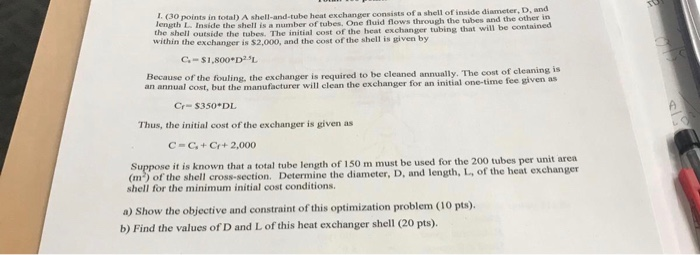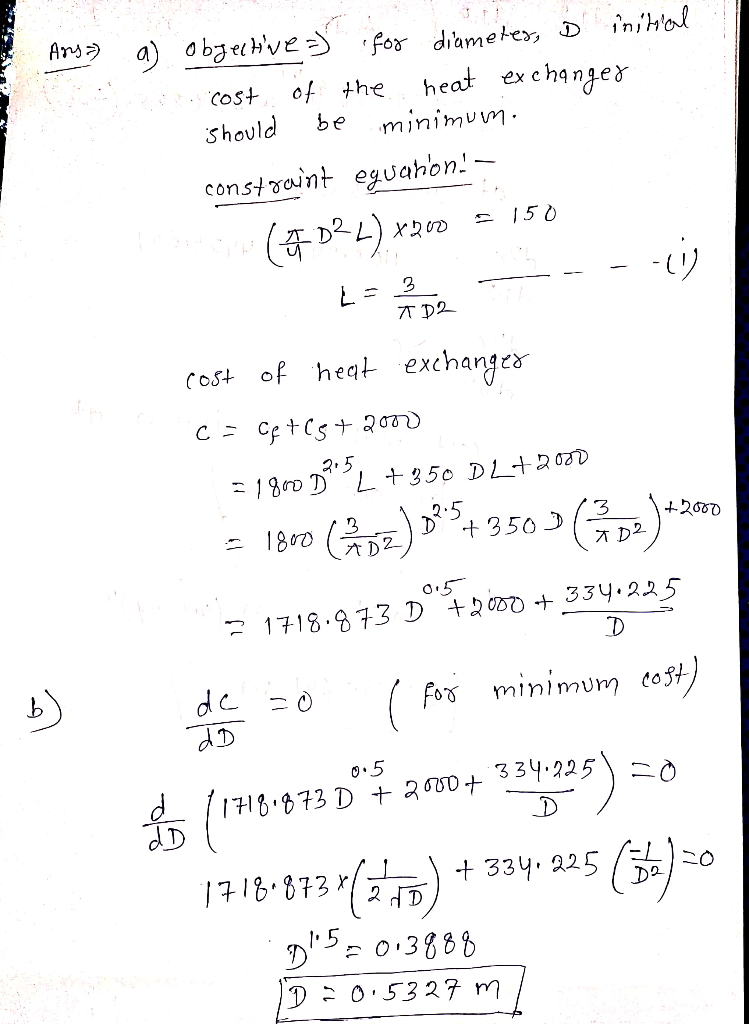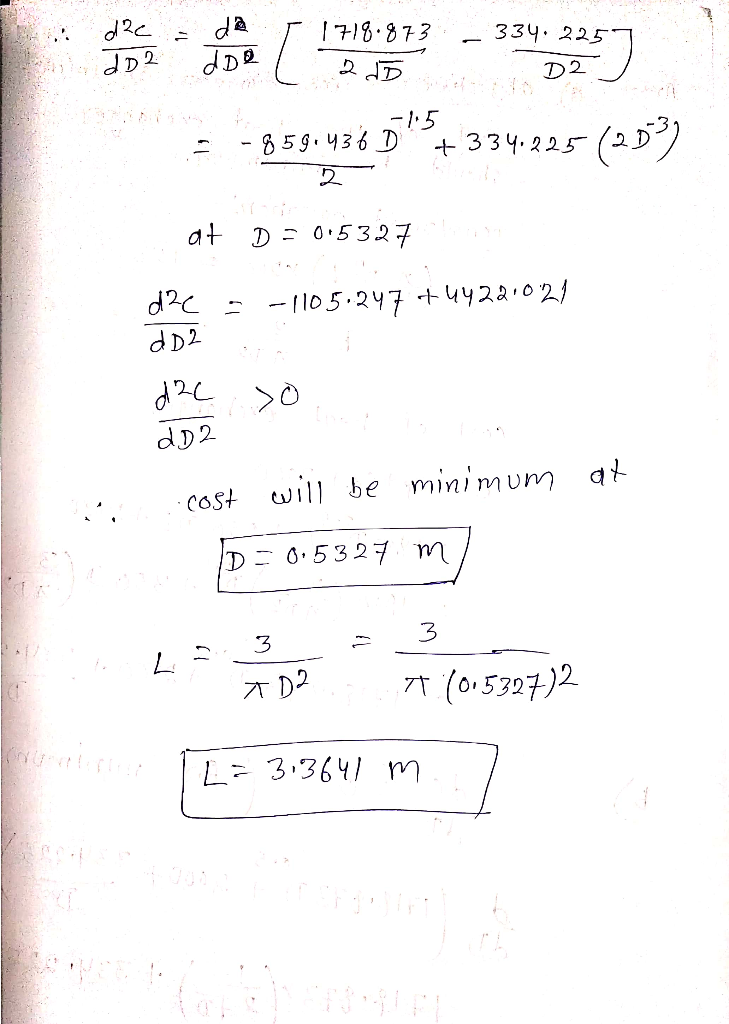Homework Help Question & Answers

# (30 points in total) A shell-and-tube heat exchanger consists of a shell of inside diameter, D, a...(30 points in total) A shell-and-tube heat exchanger consists of a shell of inside diameter, D, and length I the shell outside the tubes. within the exchanger is \$2,000, and the cost of the shell is given by f tubes. One fluid flows through the tubes and the other in ned I is a number o The initial cost of the heat exchanger tubing that will be contai G-si ,800+D2.5L Because of the fouling, the exchanger is required t an annual cost, but the manufacturer will clean the exchanger for an initial one-time fee given as be cleaned annually. The cost of cleaning is Cr-\$350 DL Thus, the initial cost of the exchanger is given as С-С,+Cr+ 2,000 e it is known that a total tube length of 150 m must be used for the 200 tubes per unit area (m2) shell for the minimum initial cost conditions. of the shell cross-section. Determine the diameter, D, and length, L, of the heat exchanger a) Show the objective and constraint of this optimization problem (10 pts) b) Find the values of D and L of this heat exchanger shell (20 pts).##### Add Answer of: (30 points in total) A shell-and-tube heat exchanger consists of a shell of inside diameter, D, a...
More Homework Help Questions Additional questions in this topic.

• #### PROBLEM #3 (30 points) A shell-and (N 20) is used to heat vegetable oil on the shell side using hot water on tethat side. is Lp- 3.0 m). Each copper tube has dimensions of 15.56-mm ID and 19.05-...

Need Online Homework Help?

Get FREE EXPERT Answers
WITHIN MINUTES
Related Questions